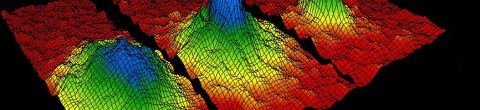Statistical MechanicsSpring, 2009

Statistical mechanics is a branch of physics that applies probability theory to the study of the thermodynamic behavior of systems composed of a large number of particles. Statistical mechanics provides a framework for relating the microscopic properties of individual atoms and molecules to the macroscopic bulk properties of materials that can be observed in everyday life. Thus it explains thermodynamics as a result of the classical and quantum-mechanical descriptions of statistics and mechanics at the microscopic level. (Source: Wikipedia)

Lectures in this Course

1. Conservation of information, energy, entropy, and temperature

Leonard Susskind discusses the study of statistical analysis as calculating the probability of things subject to the constraints of a conserved quantity. Susskind introduces energy, entropy, temperature, and phase states as they relate directly to... [more]
2. The mathematics of statistical mechanics

Leonard Susskind overviews elementary mathematics to define a method for understanding statistical mechanics.
3. The Boltzman distribution and fluctuations

Leonard Susskind reviews the Lagrange multiplier, explains Boltzmann distribution and Helmholtz free energy before oulining into the theory of fluctuations.
4. Helmholtz free energy and the partition function

Leonard Susskind explains how to calculate and define pressure, explores the formulas some of applications of Helmholtz free energy, and discusses the importance of the partition function.
5. Diatomic molecules and black hole thermodynamics

Leonard Susskind discusses the basic physics of the diatomic molecule and why you don't have to worry about its structure at low temperature. Susskind later explores a black hole thermodynamics.
6. Second law of thermodynamics

Leonard Susskind explains the second law of thermodynamics, illustrates chaos, and discusses how the volume of phase space grows.
7. Harmonic oscillators and quantum states

Leonard Susskind lectures on harmonic oscillators, quantum states, boxes of radiation and all associated computations such as wavelengths, volume, energy and temperature.
8. Magnets

Leonard Susskind lectures on a new class of systems, magnetic systems. He goes on to talk about mean field approximations of molecules in multidimensional lattice systems.
9. Phase transitions and chemical potential

Leonard Susskind picks up on magnets, phase transitions, and mean field transitions. He goes on to explain chemical potential.